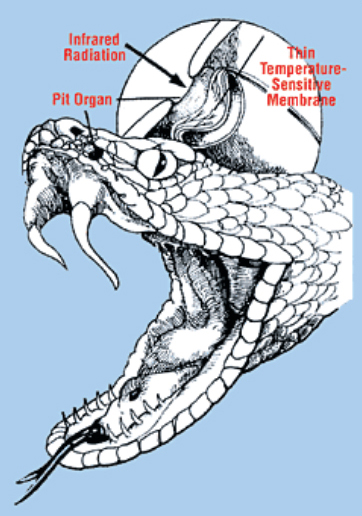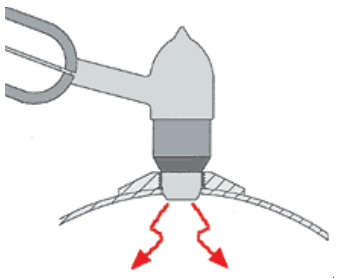# A CERTIFIED-EMISSIVITY BLACKBODY

### for Calibrating Infrared Thermometers

As a practical matter, most commercial infrared thermometers are calibrated with “homemade” blackbodies of unknown and uncertified emissivities. This design for a water-immersible certified-emissivity blackbody is intended to provide a simple and inexpensive, but accurate reference for emissivity, referenced to recognized standards and proven mathematical constructions.

Francesco Pompei, Exergen Corp.

All infrared (IR) thermometry is based on the concept of emissivity, which eventually leads mathematically to the concept of a blackbody. It is the blackbody that provides the only theoretically rigorous link between temperature and the radiated energy of precise quantifiable characteristics. Emissivity is a coefficient from 0 to 1 that compares the emitted energy of an object to that of a theoretical blackbody at the same temperature. Although not always stipulated, the mathematical concepts of emissivity and blackbody require uniform temperature.

Measuring temperature by measuring radiated energy therefore requires a reference blackbody that has known and certifiable emissivity. As a practical matter, most commercial IR thermometers are calibrated with “homemade” blackbodies of unknown and uncertified emissivities. Although the errors introduced by emissivity uncertainty are insignificant for many industrial applications, for medical use they are not negligible at all.

The design presented here for a water-immersible certified emissivity blackbody (CEBB) is intended to provide a simple and inexpensive, but accurate reference for emissivity, one that is referenced to recognized standards and proven mathematical constructions. The emissivity for this design is certified at:

0.9993< Ɛbb < 0.9997

For the maximum range of medical clinical thermometry applications (42°C target and 16°C ambient), the above uncertainty results in a maximum temperature uncertainty of ±0.01°C.

Historical Note

In 1665, Sir Isaac Newton became the first to split sunlight into colors with a prism, thus demonstrating the existence of light as radiated energy of differing wavelengths. About 135 years later, another English astronomer/scientist, William Herschel, measured the heat content of each of the colors of Newton’s spectrum and was shocked to discover that his thermometer registered the greatest heat beyond the red—in an area of the spectrum he could not see. He coined the term infrared to describe this heat energy.

Herschel demonstrated that IR heat radiation and light are simply two forms of electromagnetic energy. Our eyes see light energy because we are equipped to detect the wavelengths of visible light but not the longer IR wavelengths (see Figure 1).

One example of a special IR sensing adaptation in the animal world is the pit viper (see Figure 2), which can use the IR-sensing organs located in pits below its eyes to locate warm-blooded animals in the dark. An IR sensor, like Herschel’s thermometer and the pit viper’s organs, detects the longer wavelengths of IR energy by detecting the long-wave radiation’s heating of the measuring element.

At the turn of the twentieth century, Max Planck discovered the correct mathematical formulation of the relationship between temperature and IR radiation, thus paving the way for its use as a non-contact way to measure temperature. An unanticipated result of Planck’s work was the discovery of quantum physics, arguably the century’s most important scientific development.Figure 2. Members of the pit viper family of snakes use their infrared sensing pit organs below their eyes to locate warm-blooded prey

Recent advances in the technology of IR temperature measurement have stimulated the development of devices billions of times more sensitive than Herschel’s thermometer. Medical applications of this technology have enabled the design of devices capable of making non-invasive measurements at the temporal artery and at other body sites where speed and accuracy are required.

The Principles of Emissivity

Emissivity is a surface characteristic that determines how well an object’s temperature can be measured by an IR device. Along with background thermal radiation, emissivity is the primary source of error in IR temperature measurement. Simply stated, emissivity is the opposite of reflectivity. A perfect mirror has a reflectivity of unity and

an emissivity of zero. A perfect blackbody has an emissivity of unity and a reflectivity of zero. In actuality, all real bodies (including human ones) have an emissivity between these two limits.

Mirrors figure prominently in any discussion of heat radiation and emissivity. Since heat and light radiation behave in much the same way, we can use what we see with our eyes as examples of what the IR instrument sees. When you look in the mirror, you see only reflections and nothing of the mirror itself. If the mirror is perfect, it has 100% reflectivity. Because it reflects everything, it emits nothing. For this condition, the emissivity is zero.

If we consider an imperfect mirror, the eye then sees mostly reflection but also some of the imperfections on the mirror surface.

If, for example, we saw 90% of the mirror as a perfect reflector and 10% as imperfections, 90% of the mirror would reflect; the remaining 10% would emit. Therefore, the emissivity equals 0.1 (see Figure 3). Now consider the exact opposite of a perfect mirror—a perfect emitter. The eye looks at a perfect emitter and sees no reflection at all, only the emitting surface. Since 100% of the surface emits, and 0% reflects, the emissivity equals 1.0. This type of object is called a blackbody (see Figure 4).

And, finally, consider a good emitter. The eye sees a small amount of reflecting interspersed with a large amount of emitting. If, for example, 10% of the surface reflected instead of emitting, the emissivity would equal 0.9 (see Figure 5). Accordingly, we can state the following rule of emissivity: The emissivity of the surface is simply the percentage of the surface that emits. The remaining percentage of the surface reflects.

The Mathematics of Emissivity

Emissivity is derived from the basic physics of thermal radiation, in which the mathematical description of the energy distribution follows a formula called the Planck function:

where:

q = radiated energyat a given wavelength

Ɛ = emissivity

T = absolute target temperature

= wavelength

h, c, K, and e  = various physical constants

The Planck function integrates to the more familiar Stefan Boltzmann equation:

where:

It is important to note that if the radiation being measured does not extend over all wavelengths, the exact form of the Stefan Boltzmann equation does not strictly apply. An approximate description based on the integrated Planck function is:

where: x ≠ 4

Accordingly, corrections for spectral effects must be checked before using the Stefan Boltzmann equation if the detection system incorporates filters that restrict the wavelengths measured. Equation 4 gives the results of calculations of the spectral effect shift for several common wavelength bands, normalized to a 100°F (37.8°C) target:

spectral factor =

As is evident from Table 1, the spectral correction must be included if calibrations are to be checked over a wide target temperature range, and the spectral characteristics must be known.

To account for the effect of reflected energy, Kirchoff’s law is

applied:

emitted + reflected + transmitted energy = 1

or

Since for opaque surfaces  = 0, then  Ɛ + ρ = 1. For the case of a blackbody aperture, the reflected energy has as its source the ambient radiation field surrounding the aperture. For poor blackbodies (Ɛ <0.99) the reflected ambient energy cannot be neglected. The source of the reflected ambient

energy for the case of a probe inserted into the blackbody is

the probe itself (see Figure 6). Accordingly, if an emissivity

correction is required, the probe geometry and temperature must be known.Figure 6. Because of radiation emitted by the probe into the blackbody, its geometry and temperature should be known for any small corrections

Linear Approximation

For convenience in analysis, a linear approximation of Equation 2 may be used when comparing emissivities if the temperature is held within a narrow range. Deriving the relationship:

If the target blackbody temperatures are held to within 0.5°C, the non linearity is:

= 0.99999935

With the linearity assumption valid to one part in 106

for small temperature differences, linear forms can be used to conveniently perform the calculations for emissivity variations (see Figure 7).

Accordingly, the change in emissivity of a blackbody, or from one blackbody to another at the same temperature, may be given as:

Where:

T’= effective temperature of radiation at the probe in the aperture, measured by the same probe at the same temperatureTA. Note the importance of instrument probe temperature, since as TA -> TBB, emissivity change cannot be evaluated.

Certified Blackbody Emissivity Analysis

The spherical cavity shape has been selected for certification, since it is the only geometry for which an exact analytical solution can be calculated for emissivity .

Following the method of Bedford, the effective emissivity of spherical CEWIBB-1 may be simply looked up in . For the ratio Ra/Rs (aperture-to-sphere radius) of 0.1 for the actual device, and wall emissivity of 0.9 (nominal value for the anodized aluminum walls), the effective emissivity is 0.9997, which we can term the “nominal” value from which errors can be assessed to provide a tolerance range:

The emissivity of the anodized walls of the sphere has been

estimated by  and by test (using a reflective cup technique ) to lie in the range 0.9 + 0.02, 0.9 – 0.1. Accordingly, the emissivity can be immediately interpolated from equation 8 as:

All other errors due to departures from spherical geometry, and from the small seam between hemispheres, are negligible since the area ratio is negligible (no proof given). Errors due to non-uniformity of the temperature of the water bath used to ensure that the entire sphere is at the same temperature are independent of the sphere’s properties, but will be on the same order as the non-uniformities, i.e., very small. If non-uniformities are maintained to <0.01°C, apparent emissivity error can be evaluated by equation 7:

In preparing an emissivity certification of other devices from the master CEWIBB-1, the water bath must be checked for uniformity, and the cross certification can be no more accurate than the temperature non-uniformity. We have described a design for an inexpensive, certified-emissivity blackbody that is both accurate and traceable by recognized

standards and mathematics.

References

1. Theory and Practice of Radiation Thermometry. 1988. D.P.

Dewitt and G.D. Nutter Eds., Wiley & Sons.

2. M.Q. Brewster. 1992. Thermal Radiative Transfer & Properties. Wiley & Sons.

3. F. Pompei. U.S. Patent No. 4,636,091.